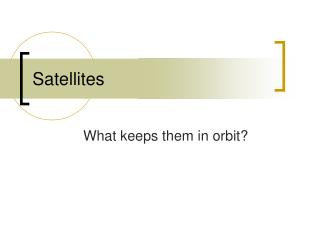# Satellites - PowerPoint PPT PresentationDownload PresentationSatellites

Satellites
Download Presentation## Satellites

- - - - - - - - - - - - - - - - - - - - - - - - - - - E N D - - - - - - - - - - - - - - - - - - - - - - - - - - -
##### Presentation Transcript

1. Satellites What keeps them in orbit?

2. Satellites • A satellite is any projectile given a large enough velocity so its path follows the curvature of the earth.

3. How fast is the earth moving? • The earth moves 8000 m in the “X” direction and 4.9 m in the “Y” direction every second. • Therefore, if a projectile moves equal or greater than that speed it will follow the curvature of the earth (it will never collide with earth)

4. What makes a satellite go? • All objects in circular motion must have a force. • The centripetal force (inward force) acting on a satellite is gravity. • Gravity pulls any satellite towards the center of the earth. • Gravity must equal centripetal force in order for satellites to orbit • What happens if its less? greater?

5. Facts about satellites • As the distance from earth increase: • Radius increase • Force of gravity decreases • Acceleration decreases • Result = required velocity is less

6. Satellite problem constants • Radius of the earth = 6.38 x 106 m • Mass of the earth = 5.97 x 1024 kg • Remember…the radius of a satellite is the height above the surface of the earth plus the radius of the earth.

7. Satellite Practice Problems #1 • Find the altitude of a satellite orbiting earth if it is moving at 6,900 m/s and the pull of gravity is 5.69 m/s2 • Answer: a = v2 r • Solve for “r”

8. Satellite Practice Problems #1 • R = (6,900 m/s)2 5.69 m/s2 • R = 8.4 x 106 m - 6.38 x 106 m • R = 2.02 x 106 m

9. Satellite Practice Problems #2 • Find the velocity needed to keep a satellite orbiting if it is accelerating at a rate of 8.2 m/s2 and it is 500 km above the surface of the earth. • Answer: a = v2 r • v2 = a x r …v = (sqrt)8.2 x 6370000.5m • v = 7227.3 m/s